# Scalar Valued Function in SQL Server

## Scalar Valued Function in SQL Server with Examples

In this article, I am going to discuss the user-defined Scalar Valued Function in SQL Server with examples. Please read our previous article, where we discussed Stored Procedures in SQL Server. At the end of this article, you will understand what is a Scalar function in SQL Server and how to create and use the SQL Server Scalar function with examples. Before understanding the Scalar Function in SQL Server, let us first understand what is a function and the different types of functions available in SQL Server.

##### What is a function in SQL Server?

A function in SQL Server is a subprogram that is used to perform an action such as complex calculation and returns the result of the action as a value. There are two types of functions in SQL Server, such as

1. System Defined Function
2. User-Defined Function

The functions which are already defined by the system and ready to be used by the developer are called system-defined functions whereas if the function is defined by the user or developer then such types of functions are called the user-defined function.

Some functions take parameters; do some processing and returning some results back. For example SELECT SQUARE(3)
Some functions may not take any parameters, but returns some result, for example, SELECT GETDATE()
So we can say that a function can have a parameter that is optional but a function should always return a value that is mandatory.

##### Types of User-Defined Function:

In SQL Server, we can create three types of User-Defined Functions, such as

1. Scalar Valued Functions
2. Inline Table-Valued Functions
3. Multi-Statement Table-Valued Functions

In this article, we are going to discuss SQL Server Scalar Valued Function in detail with Examples, the rest two user-defined functions are going to be discussed in our next article.

##### SQL Server Scalar Valued Functions

The user-defined function which returns only a single value (scalar value) is known as the Scalar Valued Function. Scalar Value Functions in SQL Server may or may not have parameters that are optional but always return a single (scalar) value which is mandatory. The returned value which is return by the SQL Server Scalar Function can be of any data type, except text, ntext, image, cursor, and timestamp. Following is the syntax to create a User-Defined Scalar Value Function in SQL Server.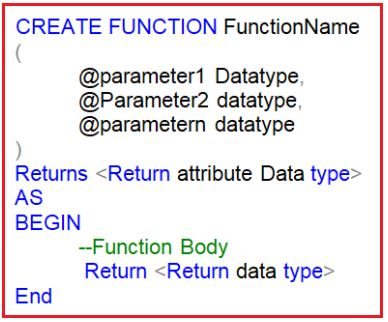As you can see in the above image, we can use Create Function followed by the function name Statement to create a user-defined function in SQL Server. Then we need to specify the input parameters. It is optional. Then we need to specify the return value data type i.e. going to be returned from the function body by using the Returns followed by the return data type statement. This is mandatory. Then we need to write the function body in between the AS BEGIN and the AND block. From the function body, at some point, we need to write the Return statement followed by the return value. This is mandatory.

##### Syntax for calling a Function in SQL Server:

SELECT dbo.<FunctionName>(Value)
When calling a scalar user-defined function we must specify the two-part name i.e. owner name and function name. The dbo stands for the database owner name. We can also invoke a scalar function in SQL Server using the complete three-part name i.e. database name. Owner name and function name.

##### Examples: SQL Server Scalar Valued User-Defined Functions

Let us understand the SQL Server Scalar User-Defined Function with some Examples.

###### Example1: Create a Scalar Function in SQL Server which will return the cube of a given value.

The function will an integer input parameter and then calculate the cube of that integer value and then returns the result. Here, the result produced will be in Integer. So, while creating the function, we need to specify the Returns data type as INT. The following function exactly does the same.

```CREATE FUNCTION SVF1(@X INT)
RETURNS INT
AS
BEGIN
RETURN @X * @X *@X
END
```

Once you created the function, as it is a database object, so it will be physically created inside the database with the following folder location. As it is a Scalar function, so it is created inside the Scalar-Valued Function folder as shown in the below image.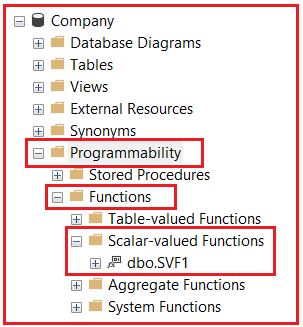To execute the above function call it like below
SELECT dbo.SVF1(5)
Output: 125

###### Example2: Write a Scalar Function to get the date difference.

Let us now create a function that will calculate and returns the age of an employee. To compute the age we require the date of birth. So, let’s pass the date of birth as a parameter. So the user-defined CalculateAge() function returns an integer and accepts date parameter.

```CREATE FUNCTION CalculateAge
(
@DOB DATE
)
RETURNS INT
AS
BEGIN
DECLARE @AGE INT
SET @AGE = DATEDIFF(YEAR, @DOB, GETDATE())-
CASE
WHEN (MONTH(@DOB) > MONTH(GETDATE())) OR
(MONTH(@DOB) = MONTH(GETDATE()) AND
DAY(@DOB) > DAY(GETDATE()))
THEN 1
ELSE 0
END
RETURN @AGE
END
```

Calling the above function:
SELECT dbo.CalculateAge (’02/29/1988′)
SELECT dbo.CalculateAge (’02/29/1988′) AS AGE

##### Scalar Valued Function in Select Clause:

The User Defined Scalar Valued Function can also be used in the select clause of an SQL Query in SQL Server. To understand the above points we are going to use the following Employee table.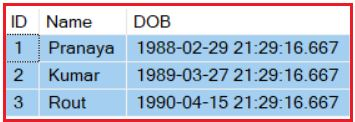Please use the following SQL Script to create and populate the Employee table with the required sample data.

```-- Create Table Employee
CREATE TABLE Employee
(
ID INT PRIMARY KEY,
Name VARCHAR(50),
DOB DATETIME
)
GO

-- Populate Employee table with some test data
INSERT INTO Employee(ID, Name, DOB) VALUES(1, 'Pranaya', '1988-02-29 21:29:16.667')
INSERT INTO Employee(ID, Name, DOB) VALUES(2, 'Kumar', '1989-03-27 21:29:16.667')
INSERT INTO Employee(ID, Name, DOB) VALUES(3, 'Rout', '1990-04-15 21:29:16.667')
```

Let’s see how to use the SQL Server Scalar Function i.e. CalculateAge function in the Select clause of an SQL Query. As you can see in the below query, in the select clause we call the CalculateAge function by passing the DOB as an input parameter. The CalculateAge function takes the DOB value and returns the Age.

```SELECT ID, Name, DOB, dbo.CalculateAge(DOB) AS Age
FROM Employee
```

Once you execute the above query, you will get the following output.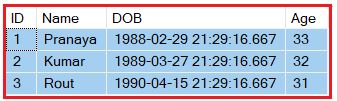##### SQL Server Scalar Valued Function in Where Clause:

Let’s see how to use the user-defined Scalar Valued function (CalculateAge) in the where clause of an SQL Query. Let’s find out the details of those employees whose age is greater than 31. At the current date, we have two employees whose age is greater than 31.

```SELECT ID, Name, DOB, dbo.calculateAge(DOB) AS Age
FROM Employee
WHERE dbo.calculateAge(DOB) > 31
```

Once you execute the above query, you will get the following output.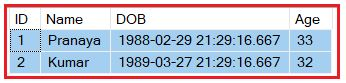If you want to alter the function then you need to use ALTER FUNCTION FuncationName statement and to delete the function, you need to use DROP FUNCTION FuncationName. To view the text of the user-defined function you need to use sp_helptext FunctionName

A stored procedure can also accept the DOB of an employee and return the age but we cannot use a stored procedure in a select clause or where clause. This is one of the differences between a function and a stored procedure. In our upcoming articles, we will discuss more differences between them.

###### Example:
```CREATE PROCEDURE spCalcualateAge(@DOB DATE)
AS
BEGIN
DECLARE @AGE INT
SET @AGE =DATEDIFF(YEAR, @DOB,GETDATE())-
CASE
WHEN (MONTH(@DOB)>MONTH(GETDATE()))OR
(MONTH(@DOB)=MONTH(GETDATE())AND
DAY(@DOB)>DAY(GETDATE()))
THEN 1
ELSE 0
END
SELECT @AGE
END

--Execute
Execute spCalcualateAge '02/29/1988'
```